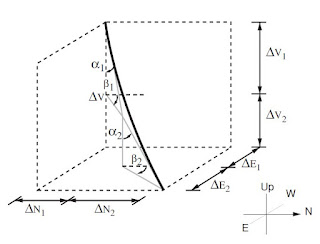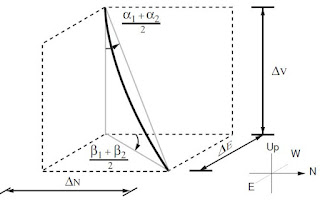# Surveying Calculations

## Surveying Calculations

### Principles of Surveying

The basic principles of surveying can be illustrated by considering the two dimensional system shown below. The position (co-ordinates) of point, B relative to the reference point A can be determined if the angle α and the distance AB is known. If the position of point A is defined as 0,0 in the X, Y co-ordinate system the position of point B can be determined by the following equations:

YB = AB Sin α
XB = AB Cos α

Hence the displacement of point B in the X and Y direction can be determined if the angle α and the linear distance between A and B are known. The position of a further point C can be determined by the same procedure. The X and Y displacement of C relative to the reference point A can be determined by adding together the X and Y displacement of Point B to A and those of Point C to B.

This process of defining the position of a point relative to a specific reference point can be continued for any number of points.

### Wellbore Surveying

This same principle as that above is applied to wellbore surveying. In the case of wellbore surveys however the procedure must include consideration of the following:
• The process must be applied in three dimensions
• The trajectory between the survey points (the path of the wellbore) is not generally a straight line
The three dimensional aspect of the problem is not a significant issue since the same process as that outlined above can be applied to the vertical displacement as well as the horizontal displacement of the survey points (stations). The procedure is described below.

The fact that the trajectory of the wellbore is not generally defined by a straight line is accommodated by assuming that the trajectory of the wellbore follows a simplified geometrical model.

The only information that is required to determine the co-ordinates of all points in the well trajectory are therefore:

#### 1- The position of the initial, reference point

(generally the Rotary Table)

#### 2- The measured depth (AHD) of the survey station

The depth of the survey station is provided by the the driller and is calculated on the basis of the length of drillstring in the wellbore and the distance between the drillbit and the survey tool.

#### 3- The direction (Degrees from North) at the survey station

The direction in which the drillbit is pointing when a survey is taken is expressed in degrees azimuth.

Azimuth is the angle in degrees (°) between the horizontal component of the wellbore direction, at a particular point, measured in a clockwise direction from the reference (generally North). Azimuth is generally expressed as a reading on a 0 - 360° (measured from North) scale.

For directional surveying, there are three azimuth reference systems :

• Magnetic north;
• True (Geographic) North;
• Grid North.
Magnetic North (MN)
This is the direction of the horizontal component of the Earth’s magnetic field lines at a particular point on the Earth’s surface. A magnetic compass will align itself to these lines with the positive pole of the compass indicating North.

True (Geographic) North (TN)
This is the direction of the geographic North Pole. This lies on the axis of rotation of the Earth. The direction is shown on maps by the meridians of longitude.

Grid North (GN)
The meridians of longitude converge towards the North Pole and South Pole, and therefore do not produce a rectangular grid system. The grid lines on a map form a rectangular grid system, the Northerly direction of which is determined by one specified meridian of longitude. The direction of this meridian is called Grid North.

For example, in the often used Universal Transverse Mercator (UTM) co-ordinate system the world is divided into 60 zones of 6 degrees of latitude, in which the central meridian defines Grid North. Grid North and True North are only identical for the central meridian. Comparison of co-ordinates is only valid if they are in the same grid system.

To be meaningful, all azimuths must be quoted in the same reference system. This is usually the Grid North system. In practice, azimuths are often measured in systems other than the Grid North system. Two conversions normally have to be applied to the measured azimuths:

• Grid Convergence : Grid convergence converts azimuth values between the Grid North and the specified True North system.
The grid convergence angle is the angle between the meridians of longitude (TN) and the North of the particular grid system (GN) at a given point. By definition, the grid convergence is positive when moving clockwise from True North to Grid North, and negative when moving anti-clockwise from True North to Grid North. The value of grid convergence depends upon location. Close to the Equator the convergence is small and it increases with increasing latitude.

• Declination: Declination converts azimuth values between the Magnetic North and True North systems. Declination is the angle between the horizontal component of the Earth’s magnetic field lines and the lines of longitude. By definition, the declination is positive when moving clockwise from True North to Magnetic North, and negative when moving anti-clockwise from True North to Magnetic North. Values ofdeclination change with time and location and those representative of the parameters at the time of drilling should be used.

#### 4- The inclination (Degrees from the vertical) of the wellbore at the survey station

The inclination of the wellbore is the angle in degrees that the wellbore is deviatedfrom the vertical.

#### 5- A mathematical models of the wellbore trajectory

Tangential Model
This model uses only the angles of inclination and direction measured at the lower survey station. The wellbore path is assumed to be tangential to these angles throughout the survey interval. The larger the angle, and the greater the survey interval, the more inaccurate the results from this model. This model is highly inaccurate and is not recommended.Tangential Model

Balanced Tangential Model
This model uses the survey data from both the upper and lower stations. The model assumes that the well path lies along two equal length, straight line segments. The inclination and direction of each segment is given by the corresponding survey station. The tangential model is therefore applied twice - once to the upper half, once to the lower half. This model approximates more closely to the probable shape of the wellbore and yields more accurate results (especially if the angles are changing rapidly).Balanced Tangential Model

Average Angle Model
In this model the inclinations and the directions at the two survey stations are averaged. The wellbore is then assumed to be one straight line over the survey interval having this average direction and inclination. This straight line path is a good approximation provided the survey interval is small, and the rate of curvature is small in the actual wellbore. This model is often used at the rig site since the calculations are fairly simple.Average Angle ModelRadius of Curvature Model